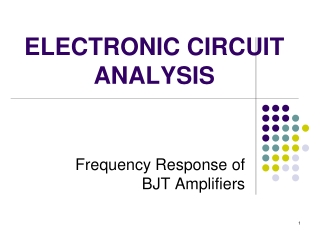DownloadDownload PresentationELECTRONIC CIRCUIT ANALYSIS

# ELECTRONIC CIRCUIT ANALYSIS

Télécharger la présentation## ELECTRONIC CIRCUIT ANALYSIS

- - - - - - - - - - - - - - - - - - - - - - - - - - - E N D - - - - - - - - - - - - - - - - - - - - - - - - - - -
##### Presentation Transcript

1. ELECTRONIC CIRCUIT ANALYSIS Frequency Response of BJT Amplifiers

2. The Decibel (dB) • A logarithmic measurement of the ratio of power or voltage • Power gain is expressed in dB by the formula: where apis the actual power gain, Pout/Pin Voltage gain is expressed by: • If av is greater than 1, the dB is +ve, and if av is less than 1, the dB gain is –ve value & usually called attenuation

3. Amplifier gain vs frequency Midband range Gain falls of due to the effects of stray capacitance and transistor capacitance effects Gain falls of due to the effects of CC and CE

4. Definition • Frequency response of an amplifier is the graph of its gain versus the frequency. • Cutoff frequencies : the frequencies at which the voltage gain equals 0.707 of its maximum value. • Midband : the band of frequencies between 10fL and 0.1fH where the voltage gain is maximum. The region where coupling & bypass capacitors act as short circuits and the stray capacitance and transistor capacitance effects act as open circuits. • Bandwidth : the band between upper and lower cutoff frequencies • Outside the midband, the voltage gain can be determined by these equations: Below midband Above midband

5. Lower & Upper Critical frequency • Critical frequency / the cutoff frequency • The frequency at which output power drops by 3 dB. [in real number, 0.5 of it’s midrange value. • An output voltage drop of 3dB represents about a 0.707 drop from the midrange value in real number. • Power is often measured in units of dBm. This is decibels with reference to 1mW of power. [0 dBm = 1mW], where;

6. Gain & frequencies • Gain-bandwidth product : constant value of the product of the voltage gain and the bandwidth. • Unity-gain frequency : the frequency at which the amplifier’s gain is 1

7. LOW FREQUENCY • At low frequency range, the gain falloff due to coupling capacitors and bypass capacitors. • As signal frequency  , the reactance of the coupling capacitor, XC - no longer behave as short circuits.

8. Short-circuit time-constant method (SCTC) • To determine the lower-cutoff frequency having n coupling and bypass capacitors: RiS = resistance at the terminals of the ith capacitor Ciwith all the other capacitors replaced by short circuits.

9. VCC = 12V R1 RC C2 30 k 4.3 k vO RS C1 0.1 F RL 1 k 2 F 100 k vS R2 RE C3 10 k 10 F 1.3 k Common-emitter Amplifier Given : Q-point values : 1.73 mA, 2.32 V  = 100, VA = 75 V Therefore, r = 1.45 k, ro =44.7 k

10. C2 vo RS C1 RC RL vs RB RE C3 Common-emitter Amplifier- Low-frequency ac equivalent circuit In the above circuit, there are 3 capacitors (coupling plus bypass capacitors). Hence we need to find 3 resistances at the terminals of the 3 capacitors in order to find the lower cut-off frequency of the amplifier circuit.

11. RinCE R1S RS RC RL RB Circuit for finding R1S Replacing C2 and C3 by short circuits

12. RoutCE R2S RC RB RS Circuit for finding R2S Replacing C1 and C3 by short circuits RL

13. RTH RC||RL RS RB RE R3S RoutCC Circuit for finding R3S Replacing C1 and C2 by short circuits

14. Estimation of L

15. RS 4.7 F 1 F vO 100  C2 C1 RL RE RC vS 75 k 43 k 22 k -VEE +VCC Common-base Amplifier Given : Q-point values : 0.1 mA, 5 V  = 100, VA = 70 V Therefore, gm = 3.85 mS, ro = 700 k r = 26 

16. vo RS C2 C1 RC RL RE vs Common-base Amplifier - Low-frequency ac equivalent circuit

17. R1S RS RE RC || RL RinCB Circuit for finding R1S Replacing C2 by short circuit

18. RoutCB R2S RS || RE RC RL Circuit for finding R2S Replacing C1 by short circuit

19. Estimation of L

20. +VCC RS C1 1 k 0.1 F C2 RB vO vS 100 k 100 F RL RE 47 k 3 k -VEE Common-collector Amplifier Given : Q-point values : 1 mA, 5 V  = 100, VA = 70 V Therefore, r = 2.6 k, ro =70 k

21. RS C1 C2 vo vs RB RE RL Common-collector Amplifier - Low-frequency ac equivalent circuit

22. Circuit for finding R1S RinCC R1S Replacing C2 by short circuit RS RB RE || RL

23. RoutCC R2S RTH = RS || RB RE RL Circuit for finding R2S Replacing C1 by short circuit

24. Estimation of L

25. VCC = 10V R1 RC C2 62 k 2.2 k vO RS C1 0.1 F RL 600 0.1 F 10 k R2 RE C3 vS 22 k 10 F 1.0 k Example Given : Q-point values : 1.6 mA, 4.86 V  = 100, VA = 70 V Therefore, r = 1.62 k, ro = 43.75 k, gm = 61.54 mS Determine the total low-frequency response of the amplifier.

26. Low frequency due to C1 and C2 C3 Low frequency due to C1 Low frequency due to C2

27. Low frequency due to C3 Low frequency due to C3